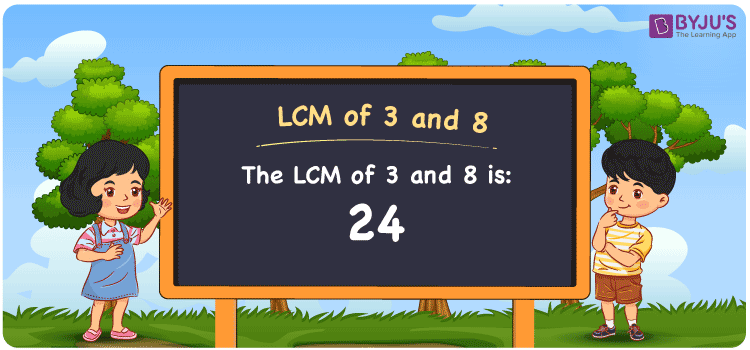# LCM of 3 and 8

LCM of 3 and 8 is 24. In mathematics, the LCM of any two numbers is the value that is evenly divisible by the two values. Among all common multiples of 3 and 8, the LCM of Two Numbers that is 3 and 8 is the smallest value. (3, 6, 9, 12, 15, 18,… ) and (8, 16, 24, 32, 40, 48, 56,… ) are the first few multiples of three and eight, respectively. Prime factorization, listing multiples, and division are the three most frequent methods for determining the LCM of 3 and 8.

## What is LCM of 3 and 8?

The answer to this question is 24. The LCM of 3 and 8 using various methods is shown in this article for your reference. The LCM of two non-zero integers, 3 and 8, is the smallest positive integer 24 which is divisible by both 3 and 8 with no remainder.## How to Find LCM of 3 and 8?

LCM of 3 and 8 can be found using three methods:

• Prime Factorisation
• Division method
• Listing the multiples

### LCM of 3 and 8 Using Prime Factorisation Method

The prime factorisation of 3 and 8, respectively, is given by:

3 = 3

4 = 2 x 2 x 2 = 23

LCM (3, 8) = 24

### LCM of 3 and 8 Using Division Method

We’ll divide the numbers (3, 8) by their prime factors to get the LCM of 3 and 8 using the division method (preferably common). The LCM of 3 and 8 is calculated by multiplying these divisors.

 2 3 8 2 3 4 2 3 2 3 3 1 x 1 1

No further division can be done.

Hence, LCM (3, 8) = 24

### LCM of 3 and 8 Using Listing the Multiples

To calculate the LCM of 3 and 8 by listing out the common multiples, list the multiples as shown below.

 Multiples of 3 Multiples of 8 3 8 6 16 9 24 12 32 15 40 18 48 21 56 24 64

The smallest common multiple of 3 and 8 is 24.

LCM (3, 8) = 24

## Video Lesson on Applications of LCM## LCM of 3 and 8 Solved Example

1. Verify the relationship between GCF and LCM of 3 and 8.

The relation between GCF and LCM of 3 and 8 is given as,

LCM(3, 8) × GCF(3, 8) = Product of 3, 8

Prime factorization of 3 and 8 is given as, 3 = (3) = 31 and 8 = (2 × 2 × 2) = 23

LCM(3, 8) = 24

GCF(3, 8) = 1

LHS = LCM(3, 8) × GCF(3, 8) = 24 × 1 = 24

RHS = Product of 3, 8 = 3 × 8 = 24

⇒ LHS = RHS = 24

Hence, verified.

## Frequently Asked Questions on LCM of 3 and 8

Q1

### If the LCM of 8 and 3 is 24, Find its GCF.

LCM(8, 3) × GCF(8, 3) = 8 × 3
Since the LCM of 8 and 3 = 24
24 × GCF(8, 3) = 24
Therefore, the GCF (greatest common factor) = 24/24 = 1.
Q2

### What are the Methods to Find LCM of 3 and 8?

The commonly used methods to find the LCM of 3 and 8 are:
Listing Multiples
Division Method
Prime Factorization Method
Q3

### What is the LCM of 3 and 8?

The LCM of 3 and 8 is 24. To find the least common multiple (LCM) of 3 and 8, we need to find the multiples of 3 and 8 (multiples of 3 = 3, 6, 9, 12 . . . . 24; multiples of 8 = 8, 16, 24, 32) and choose the smallest multiple that is exactly divisible by 3 and 8, i.e., 24.